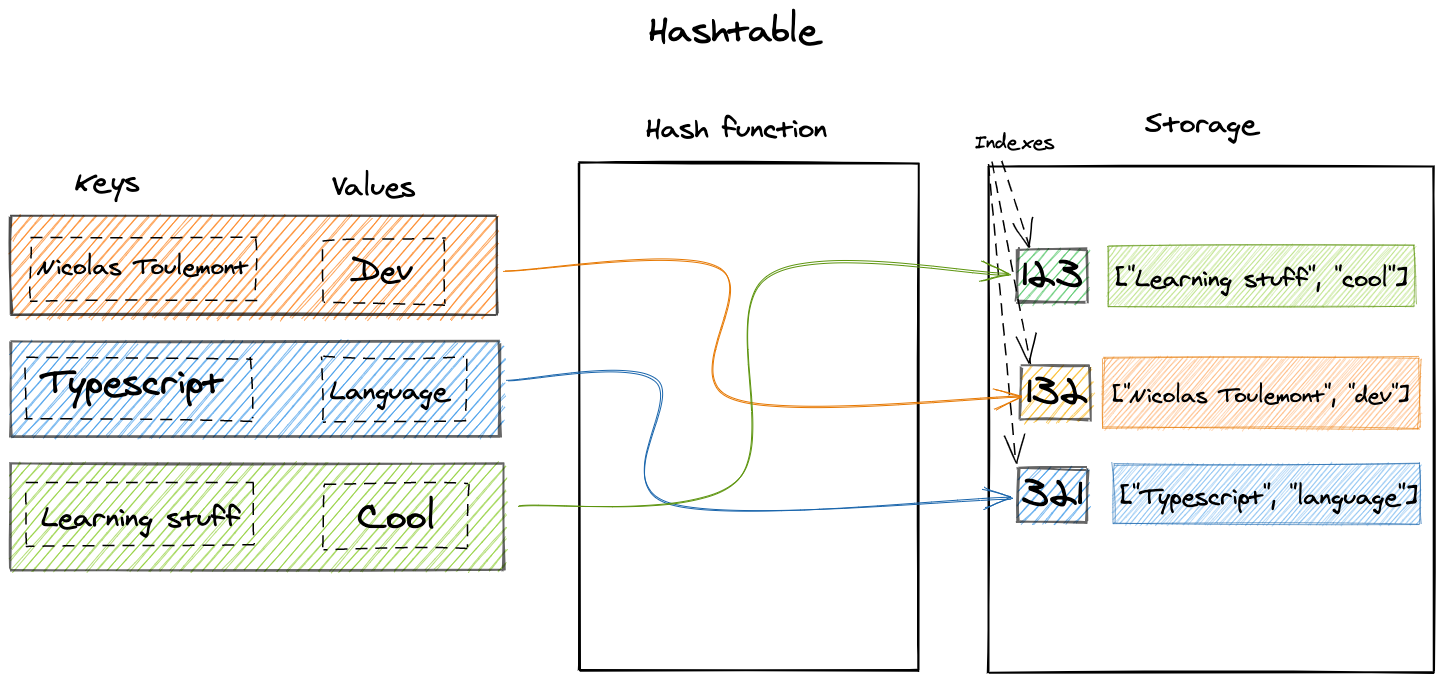# The Hashtable

Nicolas Toulemont - Dec 5th 2020
Data Structures

## What is a Hashtable ?

A hashtable is data structure allowing for key-value mapping using an associative array abstract data type. A hashtable uses a hash function to generate an index at which the key value pair will be inserted into the hashtable. Therefore, the hashing function should compute a unique and constant index for each value, but some hashing function can generate the same index for different keys. This is what is called a hash collision which we won't get into here as we will only implement a simple hashtable.### Benefits

The hashtable allow for a very efficient access to the data, as their is no need to interate on every items of the hashtable to find to target. This direct access makes it a good data structure for lookup objects in Javascript / Typescript to avoid nested loops. On each data access, only the required key is computed in order to retrieve the index.

### Practical uses in web development

Hashtable have many uses in web development, one of my favourite is a lookup object to avoid nested loops are prevent performance issues. In Javascript and Typescript, object can be uses as a very basic hashtable to store values with uniques keys. Such pattern is very helpful for query batching in the dataloader pattern to avoid n+1 issues with graphql relations.

While not implemented as a Javascript hashtable in the following example the lookup object act as one and uses the item id as key for its value in the lookup object. This lookup is then uses for direct data access in the returned array.map fonction. This allow this batchQueries function to avoid using a nested loop in the return array map function. It means that we only interate on the data and ids arrays once.

``````export async function batchQueries<T extends Document>(
model: Model<T>,
ids: Array<string>
) {
const data = await model.find({ _id: { \$in: ids } })
const lookup: Record<string, T> = data.reduce((acc: Record<string, T>, item: T) => {
acc[item.id] = item
return acc
}, {})
return ids.map((id) => lookup[id] || null)
}``````

In Javascript and Typescript, the unique key constraint of the object makes it a good candidat for basic hashtable usages such as lookup objects.

## Basic hashtable

We will now implement a hashtable using Typecript class syntax.

First we need to create a hashing function that will output the same value for the same key:

``````function hashingFn(string: string, number: number) {
let sum = 0
for (let i = 0; i < string.length; i++) {
sum += string.charCodeAt(i) * 3
}
return sum % number
}``````

Then the queue properties and initialization. This hashtable will be given a size parameter used in the hashing function and hold the data in a storage array.

``````export class HashTable<T> {
size: number
storage: Array<Array<[string, T]>>
constructor(size: number) {
this.size = size
this.storage = []
}
}``````

### Basic methods

• Insert()

The insert method first create an index for the given key and then insert the value at the given index in the storage as a [key, value] array.

``````insert(key: string, value: T) {
const index = hashingFn(key, this.size);

if (!this.storage[index]) {
this.storage[index] = [];
}
this.storage[index].push([key, value]);
}``````
• Get()

The get method is quite simple as it first compute the index for target key, get the storage value reference at the given index and then interate on the array value to return nested array index 1 (the value).

``````get(key: string) {
const index = hashingFn(key, this.size);
let arrayAtIndex = this.storage[index];
if (!arrayAtIndex) return null;

for (let i = 0; i < arrayAtIndex.length; i++) {
if (arrayAtIndex[i] && arrayAtIndex[i] === key) {
return arrayAtIndex[i];
}
}
return null;
}``````
• Remove()

The remove method does the same as the get method but then delete the nested array whose key match the given key.

``````remove(key: string) {
const index = hashingFn(key, this.size);
let arrayAtIndex = this.storage[index];
if (arrayAtIndex) {
for (let i = 0; i < arrayAtIndex.length; i++) {
arrayAtIndex[i] === key && delete arrayAtIndex[i];
break;
}
}
}``````